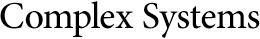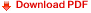## Exact Calculation of Lyapunov Exponents and Spreading Rates for Rule 40Fumio Ohi
Nagoya Institute of Technology
Gokiso-cho, Showa-ku, Nagoya 466-8555, Japan

#### Abstract

In this paper we exactly calculate the Lyapunov exponents and two kinds of spreading rates for elementary cellular automaton (ECA) rule 40. One spreading rate, simply called the spreading rate, is defined by following A. Ilachinski , and the other one originally defined in this paper is called the strict spreading rate. For the Lyapunov exponents, we use M. A. Shereshevsky's definition . For an arbitrarily given rational number between 1/2 and 1, we specifically construct a configuration having the Lyapunov exponent equal to the rational number. For rule 40, the Lyapunov exponent is equal to the spreading rate but not necessarily to the strict spreading rate. For the strict spreading rate, it is proved that for an arbitrarily given real number between 1/2 and 1, there exists a configuration having the strict spreading rate equal to the given real number. This theorem is proved by construction. These dynamical properties are observed on the set of configurations of a specific type. We formally prove that the Bernoulli measure of this set is 0, which is why these dynamical properties have not been observed in computer simulations.

https://doi.org/10.25088/ComplexSystems.19.4.323